# need help: problem in write/read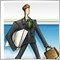5079

HI. I try it as below,

can you please check this part of code;

i have store data in "  write " part and they are true and i have the file .(have problem about time )

then i want to read them in " read " part and when i print them on chart have problem for time : the part have time parameter highlighted have problem .( i think)

```//++++++++++++++++++++++++++++++++++++++++++++++++++++++++++++++++++++++++
//++++++++
//+++++++++++++++++++++

//----------------------------------------------------------------------------------------------------------//
void OnTick(void)
{
//----------------------------------------------------------------------------------------------------------//
.
//------------------------------------------------------------------------------------------  FILE WRITE

//---
//string terminal_data_path=TerminalInfoString(TERMINAL_DATA_PATH);
//string filename=terminal_data_path+"\\MQL5\\Files\\"+"fractals.csv";
//---
ResetLastError();
{
for(int wr = 0; wr < 26; wr++)
{
//  TimeCurrent:  ", TimeToStr(TimeCurrent() - 7200, TIME_MINUTES|TIME_SECONDS),TimeToString
}
//--- close the file
PrintFormat("Data is written, %s file is closed",filenameWRITE_BUY);

}
else Print("PROBLEM TO SEND TXT FILE! Error = ",GetLastError());
//----------------------------------------------------------------------//

ResetLastError();
//--- open the file

{
PrintFormat("File path: %s\\Files\\",TerminalInfoString(TERMINAL_DATA_PATH));

//  TimeCurrent:  ", TimeToStr(TimeCurrent() - 7200, TIME_MINUTES|TIME_SECONDS),TimeToString
int wr1 = 0;
//for(int wr1 = 0; wr1 < 26; wr1++)
/*
{
wr1++;
continue;
}
*/

{
}

//--- close the file
}
//---

//----------------------------------------------------------------------------------------------------------//```

*** )   " PROBLEM IS IN ' TIME ' WRITE~READ  :"

can you please check it to see what is wrong .

thank you.Moderator
32153

What is the problem?

Telling us that you have a problem, tells us nothing.

Explain what you mean by "problem"5079

i run it on strategy tester , as you see the reading time is 2 hour less than the stored in write.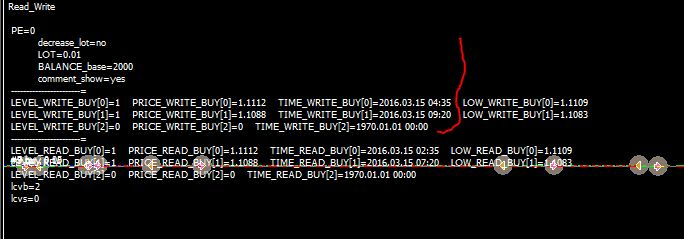and if remove the "7200" in the first highlighted line time wll be the current time as below picture:

in real time chart as below: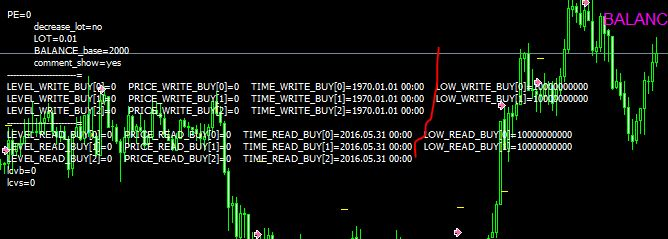also in strategy tester as below: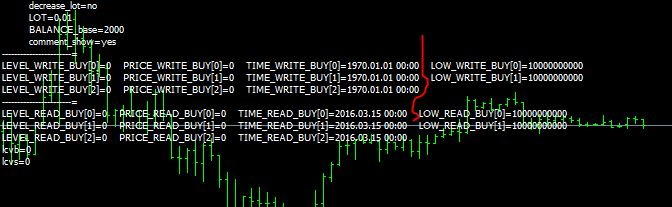----------------------------------------------------------------------------------------------------------------------------------------------------

2-) the csv file :

3th column is time : ( is look blank )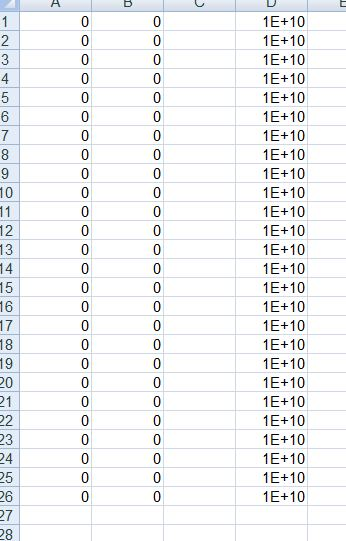25836

Don't use a spreadsheet, that's just adding another layer of misdirection. Show the file contents, command line/type or notepad.5079

problem solved: in first highlighted line :

`(TimeToString(TIME_WRITE_BUY[wr] , TIME_DATE|TIME_SECONDS))`